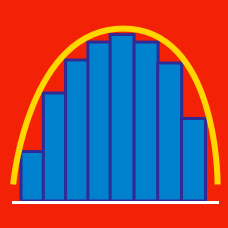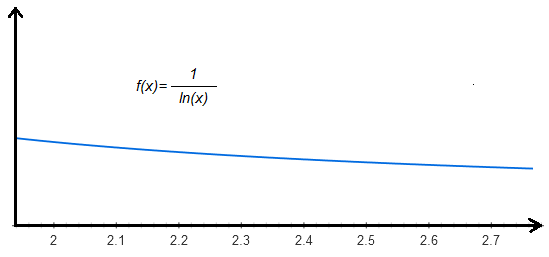Calculus

# Numerical Approximation of Integrals: Level 4 Challenges

Using the Left-hand Rectangular Approximation Method and 11 rectangles, approximate

$\large \int_{0}^{1}x^3dx .$

Give your answer to 4 decimal places.

\begin{aligned} S_{1}&=&\sqrt{1887}+\sqrt{1888}+\cdots+\sqrt{2013}+\sqrt{2014} \\ \\ \ S_{2}&=&\sqrt{1888}+\sqrt{1889}+\cdots+\sqrt{2014}+\sqrt{2015} \\ \\ I&=&\int_{1887}^{2015}\!\sqrt{x}\,\mathrm{d}x \end{aligned}

What can you say about the relative values of $S_{1}$, $S_{2}$, and $I$?

$\large \begin{cases} {f(2013) = 3} \\ {f(2015) = 1} \\ {f(2017)=5} \\ {f(2019)=2015} \\ \end{cases}$

Given that a cubic polynomial $f(x)$ that satisfy the system of equations above, find the value of $\displaystyle \int_{2013}^{2017} f(x) \, dx$.

For all cubic polynomials $f(x)$, what positive value of $k$ makes the following statement true?

$\int_{-1}^{1}f(x)dx = f(-k) + f(k)$

Bonus question: What is this method of approximation known as?

$\bigg \lfloor \;10 \times \displaystyle \int_2^e \! \frac {1}{\ln(x)} \, \mathrm{d}x\;\bigg \rfloor = \ ?$

Details and Assumptions:

• You may use the following approximations: $e \approx 2.718$, and $\ln(2) \approx 0.693$, and use the following graph of $f(x)= \frac {1}{\ln(x)}$.×

Problem Loading...

Note Loading...

Set Loading...